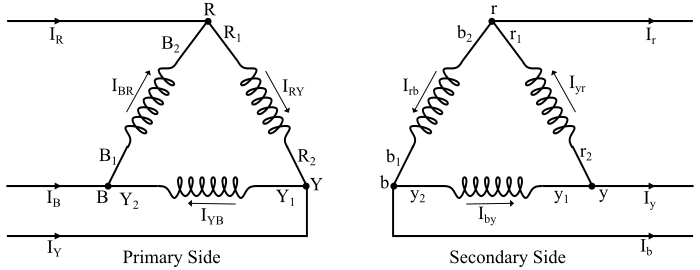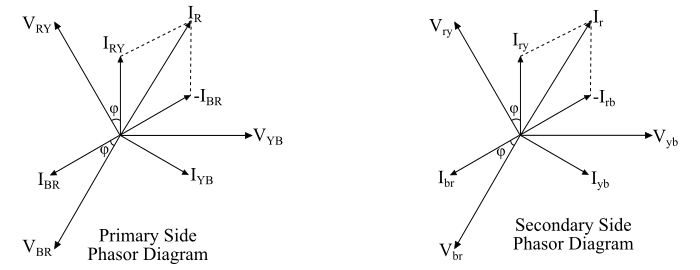# What is Delta-Delta Connection of Transformer?

The delta-delta connection of the primary and secondary windings of a three-phase transformer is shown in the figure. Here, the secondary winding r1r2 corresponds to the primary winding R1R2, and the terminals R1 and r1 have same polarity. Also, the polarity of terminal 𝐫 connecting the r1 and b2 is the same as that of R connecting R1 and B2.The phasor diagram is drawn for the usual case of lagging power factor load. Here, the magnetising current and voltage drops in the impedances have been neglected. Under balanced condition, the line currents are √3 times of the phase currents and lags behind the phases currents. In case of the delta-delta connection, the corresponding line voltages and phase voltages are same in magnitude on both primary and secondary windings.The secondary line voltages Vry, Vyb and Vbr are in phase with the primary line voltages VRY, VYB and VBR and their voltage ratios are given as follows −

$$\mathrm{\frac{𝑉_{𝑅𝑌}}{𝑉_{𝑟𝑦}}=\frac{𝑉_{𝑌𝐵}}{𝑉_{𝑦𝑏}}=\frac{𝑉_{𝐵𝑅}}{𝑉_{𝑏𝑟}}=𝐾}$$

And when the magnetising currents are neglected, the current ratios are

$$\mathrm{\frac{𝑉_{𝑅𝑌}}{𝑉_{𝑟𝑦}}=\frac{𝑉_{𝑌𝐵}}{𝑉_{𝑦𝑏}}=\frac{𝑉_{𝐵𝑅}}{𝑉_{𝑏𝑟}}=\frac{1}{𝐾}}$$

There is no phase shift in case of a delta-delta transformer and no problems with unbalanced loads or harmonics.